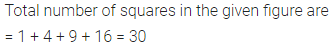ML Aggarwal Class 6 Solutions Chapter 10 Basic Geometrical Concept Objective Type Questions for ICSE Understanding Mathematics acts as the best resource during your learning and helps you score well in your exams.

## ML Aggarwal Class 6 Solutions for ICSE Maths Chapter 10 Basic Geometrical Concept Objective Type Questions

Mental Maths
Question 1.
Fill in the blanks:
(i) There is exactly one line passing through ……….. distinct points in a plane.
(ii) Two different lines in a plane either ……….. at exactly one point or are parallel.
(iii) The curves which have different beginning and end points are called ……….. curves.
(iv) A curve which does not cross itself at any point is called a ……….. curve.
(v) A simple closed curve made up entirely of line segments is called a ………..
(vi) A line segment formed by joining two non- adjacent vertices of a polygon is called its ………..
(vii) A quadrilateral has ……….. diagonals.
(viii) A lines segment has a ……….. length.
Solution:Question 2.
Fill in the blanks with correct word(s) to make the statement true.
(i) Radius of a circle is one-half of its ………..
(ii) A radius of a circle is a line segment with one end point
at ……….. and the other end-point on
(iii) A chord of a circle is a line segment with its end points ………..
(iv) A diameter of a circle is a chord that ……….. the centre of the circle.
(v) All radii of a circle are ………..
Solution: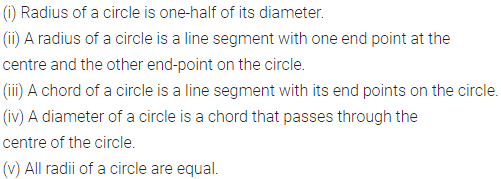Question 3.
State whether the following statements are true (T) or false (F):
(i) The line segment AB is the shortest route from A to B.
(ii) A line cannot be drawn wholly on a sheet of paper..
(iii) A line segment is made of infinite (uncountable) number of points.
(iv) Two lines in a plane always intersect.
(v) Through a given point only one line can be drawn.
(vi) Two different lines can be drawn passing through two distinct points.
(vii) Every simple closed curve is a polygon.
(viii)Every polygon has atleast three sides.
(ix) A vertex of a quadrilateral lies in its interior.
(x) A line segment with its end-points lying on a circle is called a diameter of the circle.
(xi) Diameter is the longest chord of the circle.
(xii) The end-points of a diameter of a circle divide the circle into two points, each part is called a semi-circle.
(xiii) A diameter of a circle divides the circular region into two parts, each part is called a semi-circular region.
(xiv) The diameter’s of a circle are concurrent the centre of the circle is the point common to all diameters.
(xv) Every circle has unique centre and it lies inside the circle.
(xvi) Every circle has unique diameter.
Solution: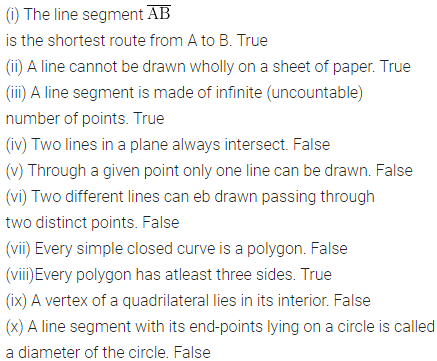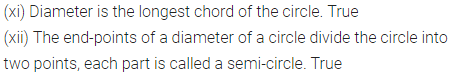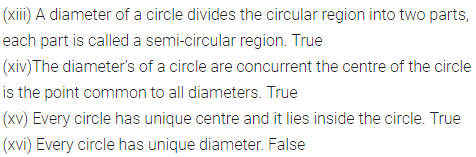Multiple Choice Questions
Choose the correct answer from the given four options (4 to 20):
Question 4.
Which of the following has no end points?
(a) a line
(b) a ray
(c) a line segment
(d) none of these
Solution:Question 5.
Which of the following has definite length?
(a) a line
(b) a ray
(c) a line segment
(d) none of these
Solution: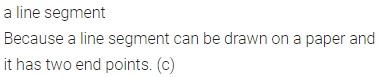Question 6.
The number of points required to name a line if
(a) 1
(b) 2
(c) 3
(d) 4
Solution:Question 7.
The number of lines that can be drawn through a given point is
(a) 1
(b) 2
(c) 3
(d) infinitely many
Solution:Question 8.
The number of lines that can be drawn passing through two distinct points is
(a) 1
(b) 2
(c) 3
(d) inifinitely many
Solution: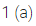Question 9.
The maximum number of points of intersection of three lines drawn in a plane is
(a) 1
(b) 2
(c) 3
(d) 6
Solution:Question 10.
The minimum number of points of intersection of three lines drawn in a plane is
(a) 0
(b) 1
(c) 2
(d) 3
Solution:Question 11.
In the given figure, the number of line segment is
(a) 5
(b) 10
(c) 12
(d) 15
Solution: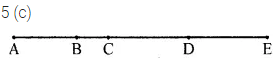Question 12.
In a polygon with 5 sides, the number of diagonals is
(a) 3
(b) 4
(c) 5
(d) 10
Solution: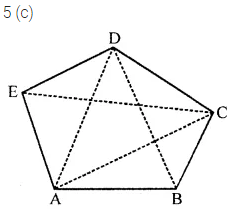Question 13.
The number of lines passing through 5 points such that no three of them are collinear are
(a) 10
(b) 5
(c) 8
(d) 20
Solution: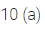Question 14.
In context of the given figure, which of the following statement is correct ?
(a) B is not a point on segment $$\overline{\mathrm{AC}}$$
(b) B is the initial point of the ray $$\overrightarrow{\mathrm{AD}}$$
(c) D is a point on the ray $$\overrightarrow{\mathrm{CA}}$$
(d) C is a point on the ray $$\overrightarrow{\mathrm{BD}}$$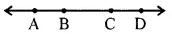Solution:Question 15.
The figure formed by two rays with same initial point is known as
(a) a line
(b) a line segment
(c) a ray
(d) an angle
Solution: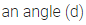Question 16.
In the given figure, the number of angles is
(a) 3
(b) 4
(c) 5
(d) 6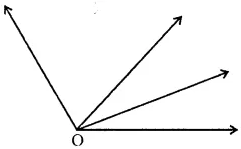Solution: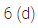Question 17.
Which of the following statements is false?
(a) A triangle has three sides
(b) A triangle has three vertices
(c) A triangle has three angles
(d) A triangle has two diagonals
Solution: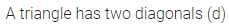Question 18.
Which of the following statements is false?
(a) A quadrilateral has four sides and four vertices
(b) A quadrilateral has four angles
(c) A quadrilateral has four diagonals
(d) A quadrilateral has two diagonals
Solution: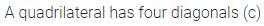Question 19.
By joining any two points of a circle, we obtain its
(b) chord
(c) diameter
(d) circumference
Solution: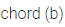Question 20.
If the radius of a circle is 4 cm, then the length of its diameter is
(a) 2 cm
(b) 4 cm
(c) 8 cm
(d) 16 cm
Solution: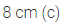Higher Order Thinking Skills (Hots)
Question 1.
Can a sector and segment of a circle coincide? If so, name it.
Solution: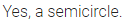Question 2.
In the given figure, find:
(i) the number of triangles pointing up.
(ii) the total number of triangles.Solution:Question 3.
In the given figure, find the total number of squares.Solution: